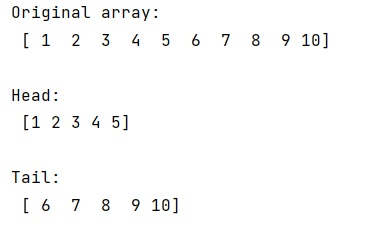# Is there a head and tail method for NumPy array?

Submitted by Pranit Sharma, on February 14, 2023

NumPy is an abbreviated form of Numerical Python. It is used for different types of scientific operations in python. Numpy is a vast library in python which is used for almost every kind of scientific or mathematical operation. It is itself an array which is a collection of various methods and functions for processing the arrays.

Let's understand whether there is a head and tail method in numpy. If not, then what is the procedure of taking some elements from the beginning and end of a numpy array.

NumPy does not support any methods named head() and tail(), but we can access the first and last n rows similar to head and tail. We can get these elements using the slicing approach.

Let us understand with the help of an example,

## Python code to demonstrate the example for getting the head and tail elements from a numpy array

```# Import numpy
import numpy as np

# Creating a numpy array
arr = np.array([1,2,3,4,5,6,7,8,9,10])

# Display original array
print("Original array:\n",arr,"\n")

# Getting first 5 elements of array

# Display the result of head

# Getting last 5 elements of array
tail = arr[5:]

# Display the result of tail
print("Tail:\n",tail,"\n")
```

Output: